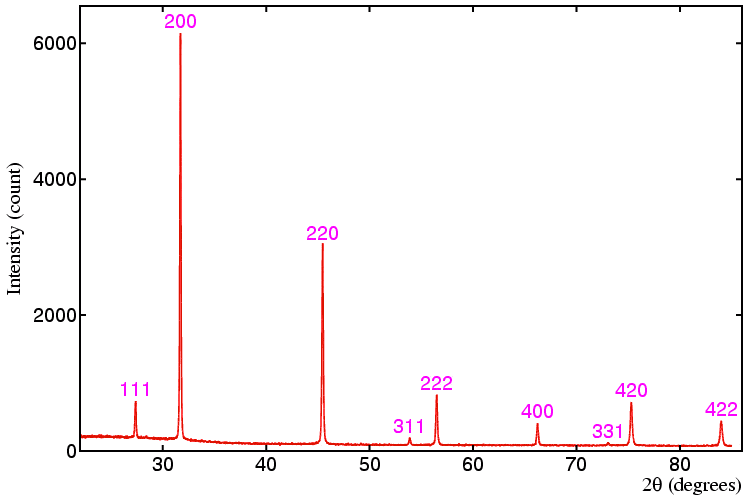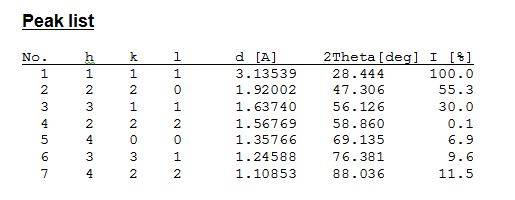# How to interpret the powder diffraction?

Hi,

I have tried to understand powder diffraction "spectrum". What I do understand is that those diffraction peaks are peaks from ewalds sphere. What I don't understand is how one can get planes and (3d) base vectors out of that. I would really appreciate some help?DrDu
Generally in crystal structure analysis, you see those peaks for which a reciprocal lattice point coincides with the Ewald sphere.
In powder diffraction, you see not only the lattice points of one reciprocal lattice, but of all reciprocal lattices which can be obtained by rotation due to the statistical orientation of the grains of the powder. The smallest reciprocal vectors (=smallest scattering angles) correspond to the planes with lowest Miller indices. The other ones must then correspond to combinations whose indices.

Thanks for the answer, I guess my question was a bit unclear. What I originally meant is that how one can say just by knowing the angle and intensity that for example the first peak is 111 and second is 200. As far as I understand these angles are standard bragg diffraction angles and I don't see how to interpret anything from those (or of course with the wavelength of the radiation one can get the distance between planes).

DrDu
That's an excellent question, but I don't know no satisfactory answer. If the crystal is of low symmetry, there is no unique system of basevectors or of Miller indices. So there seems to enter a lot of convention entering the assignment of the peaks.

From the known wave length and the measured angle, you can calculate the d-spacing for each peak using Bragg's law.

For a cubic crystal, d=a/sqrt(H^2+K^2+L^2) where a is the lattice parameter, so the classical method was to make a table of 1/d^2, which is (H^2+K^2+L^2)/a^2. By comparing the values you can guess H,K, and L for each of them and find a.

These days you use a computer program to do the analysis for you.

Dear HIUk,

The method above is actually the proper way to assign hkl values to each peak.

By any chance this is a XRD phase analysis measurement for Silicon? As far as I know, one can assign a peak to a hkl value by comparing the peak position to a JCPDS card (Joint Committee of Powder Diffraction Standards). In the old days, x-ray laboratories would buy these cards compiled in books consisting of numerous volumes. Nowadays the database should be available in soft copies format stored in the computer operating the X-ray diffractometer.

The JCPDS cards are a collective XRD data of almost all elements/compounds taken from selected experiments. Below is a screenshot from my database for silicon:From your spectrum, first get the 2θ position. Next compare your position to the 2theta column in the database. Consequently, match the hkl values in the corresponding column.

However, I do notice that your data seems to diverge from my database at higher angles and that some peaks are missing. This is probably because my database has been selected to suit my sample or the fact that our experiment is setup differently. Yours might use a different card under the same element. That is if the data above is for silicon.

Pardon my mistakes, if there is any.